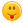# showing indentation erroe in line 9

import numpy as np
ar1=[20,30,50]
ar2=[60,40,25]
arr2 = np.ar([60,40,25])
temp=[]
temp2=[]
for element in arr2:
for i in range(3):
if ar[i]>element:
count=count+1
temp=a[i]
temp2=element

print(count)

finally, " temp=a[i]" will be an error ,you can figure this one